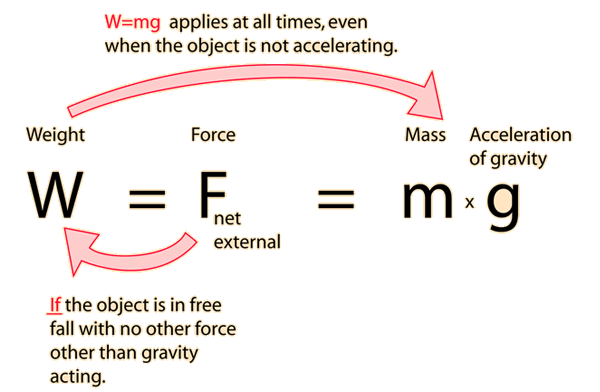# Mass and Weight

The mass of an object is a fundamental property of the object; a numerical measure of its inertia; a fundamental measure of the amount of matter in the object. Definitions of mass often seem circular because it is such a fundamental quantity that it is hard to define in terms of something else. All mechanical quantities can be defined in terms of mass, length, and time. The usual symbol for mass is m and its SI unit is the kilogram. While the mass is normally considered to be an unchanging property of an object, at speeds approaching the speed of light one must consider the increase in the relativistic mass.

The weight of an object is the force of gravity on the object and may be defined as the mass times the acceleration of gravity, w = mg. Since the weight is a force, its SI unit is the newton. Density is mass/volume.Index

 HyperPhysics***** Mechanics R Nave
Go Back

# Weight

The weight of an object is defined as the force of gravity on the object and may be calculated as the mass times the acceleration of gravity, w = mg. Since the weight is a force, its SI unit is the newton.

For an object in free fall, so that gravity is the only force acting on it, then the expression for weight follows from Newton's second law.You might well ask, as many do, "Why do you multiply the mass times the freefall acceleration of gravity when the mass is sitting at rest on the table?". The value of g allows you to determine the net gravity force if it were in freefall, and that net gravity force is the weight. Another approach is to consider "g" to be the measure of the intensity of the gravity field in Newtons/kg at your location. You can view the weight as a measure of the mass in kg times the intensity of the gravity field, 9.8 Newtons/kg under standard conditions.

Data can be entered into any of the boxes below. Then click outside the box to update the other quantities.At the Earth's surface, where g=9.8 m/s2 :

The weight of mass kg is Newtons
The weight of mass slugs is poundsThe kilogram is the SI unit of mass and it is the almost universally used standard mass unit. The associated SI unit of force and weight is the Newton, with 1 kilogram weighing 9.8 Newtons under standard conditions on the Earth's surface. However, in the US common units, the pound is the unit of force (and therefore weight).The pound is the widely used unit for commerce. The use of the pound force constrains the mass unit to an inconveniently large measuring unit called a "slug". The use of this unit is discouraged, and the use of exclusively SI units for all scientific work is strongly encouraged.
 What is a slug?
Index

 HyperPhysics***** Mechanics R Nave
Go Back

# Weightlessness

While the actual weight of a person is determined by his mass and the acceleration of gravity, one's "perceived weight" or "effective weight" comes from the fact that he is supported by floor, chair, etc. If all support is removed suddenly and the person begins to fall freely, he feels suddenly "weightless" - so weightlessness refers to a state of being in free fall in which there is no perceived support. The state of weightlessness can be achieved in several ways, all of which involve significant physical principles.

Click on any of the examples for further details.Index

 HyperPhysics***** Mechanics R Nave
Go Back